# Math Practice Topic: Transformations

 Description: Translation, rotation, reflection. Adaptive Learning Progression: Translation, then rotation, then reflection. Start using MathScore for free

## Sample Levels (out of 6)

 1 Figure out the translation components, and then where point P' ends up: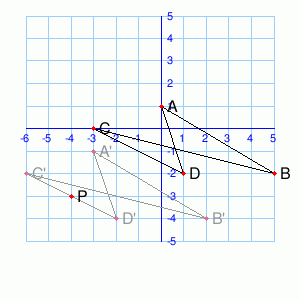Translation: ( ,) Let P = (-4, -3). P' = ( ,) 2 Figure out the translation components, and then where point P' ends up: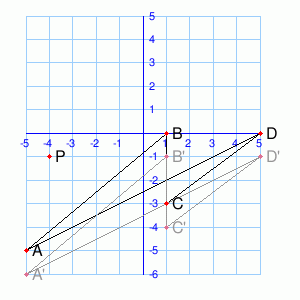Translation: ( ,) Let P = (-4, -1). P' = ( ,)

 1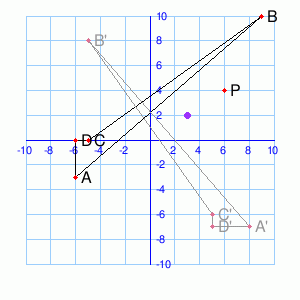The transformation ABCD → A'B'C'D' is a rotation around (3, 2) by ° Rotate P around (3, 2) by the same angle. (You may need to sketch things out on paper.) P' = ( ,) 2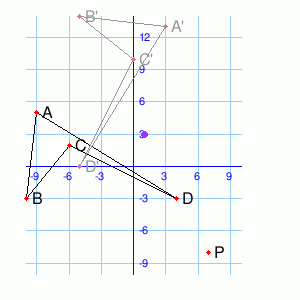The transformation ABCD → A'B'C'D' is a rotation around (1, 3) by ° Rotate P around (1, 3) by the same angle. (You may need to sketch things out on paper.) P' = ( ,)

 1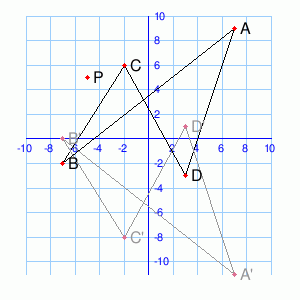Transformation ABCD → A'B'C'D' is a reflection across the line e.g. y=-3, y=x. Reflect P over the same line. (You may need to sketch things out on paper.) P' = ( ,) 2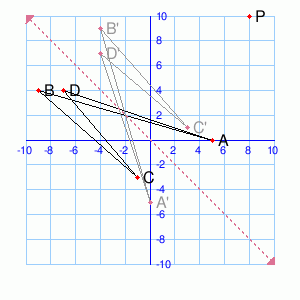Transformation ABCD → A'B'C'D' is a reflection across the line e.g. y=-3, y=x. Reflect P over the same line. (You may need to sketch things out on paper.) P' = ( ,)